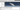9 /5/2019

# Constructing 3D Polyhedra in Abaqus using Python scripting

Colab Notebook here

If you’re into heterogeneous modelling then you might’ve come across constructing a 3D polygon with random vertices. In my context, constructing such a model was to investigate mesoscale effects in a concrete material (made up of coarse and fine aggregates). Regardless, this methodology of using Abaqus and Python to construct a polyhedra is applicable cross-discipline.#### Getting Started

To get started, you will need a set of polygon vertex coordinates and its convex hull, which you can download here.

For a bit of background on my workflow, I’ve generated random vertices for the polyhedra and determined the convex hull using Matlab (convhull function).

In this generation script, I’m reading from the csv file, which constitutes to one polyhedra dataset, then using Abaqus methods which can be determined from recording macro to programmatically create the geometry.

``````from abaqus import *
from abaqusConstants import *
import numpy as np
import os
import __main__

import section
import regionToolset
import displayGroupMdbToolset as dgm
import part
import material
import assembly
import step
import interaction
import mesh
import optimization
import job
import sketch
import visualization
import xyPlot
import displayGroupOdbToolset as dgo
import connectorBehavior

csvPath = r"/path" #path storing the polygon data
os.chdir(csvPath)

data = [];
convexHull = [];
data = np.genfromtxt('polygon1.csv', delimiter=',')
convexHull = np.genfromtxt('polygon1-hull.csv', delimiter=',')``````

#### Procedure

Once we have imported the Abaqus modules and loaded the polygon data in, we can now start drawing the 3D polygon. The general procedure is as follows:

1. Create datum points which represent each polygon vertex (Part > Datum Point)
2. Join datum points with a wire line (Shape > Wire > Point to Point) by following the convex hull configuration
3. Each wire line represents an edge, and 3 edges which forms a triangle can be converted to a face. To do this (Tools > Geometry Edit > Face > Cover Edges > select 3 edges that enclose the face)
4. Convert all wire lines into faces
5. Select all faces and convert it to a solid (Shape > Solid > From shell > Select all faces)

#### Create Vertices using Datum Points

Now, let’s have a look at the python code to script this.

``````# create coordinate reference and supress
p = mdb.models['Model-1'].Part(name='Part-1', dimensionality=THREE_D, type=DEFORMABLE_BODY)
p.ReferencePoint(point=(0.0, 0.0, 0.0))
p.features['RP'].suppress()

for item in data:
p.DatumPointByCoordinate(coords=(item, item, item))

d1 = p.datums;``````

In the code above, we created a part with the default name “Part-1”, then created a reference point to initalise a 3D space to sketch in. After that, using DatumPointByCoordinate which is a method which creates datum points, we create all the vertices from the variable data by looping through it.

#### Create Edges using Wires

Next part of the code is to loop through the newly created datum points and join them up using wire lines. To determine which datum points to join in order, we need to refer to the convex hull. The convexHull data is already assigned in the first block of code.

``````# join datum for edges
for hull in convexHull:
p.WirePolyLine(points=((d1[int(hull)+1], d1[int(hull)+1]),), mergeType=IMPRINT, meshable=ON)
p.WirePolyLine(points=((d1[int(hull)+1], d1[int(hull)+1]),), mergeType=IMPRINT, meshable=ON)
p.WirePolyLine(points=((d1[int(hull)+1], d1[int(hull)+1]),), mergeType=IMPRINT, meshable=ON)

eg = p.edges``````

p.wirePolyLine is the method to draw a wireline, and we’re joining datum points for all possible combinations for each row provided in the convex hull data. For example: A triangle is represented as (P1, P2, P3). To draw this triangle, we need a line between P1 and P2, P2 and P3 and finally P1 and P3.

An edge is automatically created for each wire line drawn, and we can access that array of edges by calling p.edges, where p is the model part variable name defined earlier.

#### Create Faces from Three Edges

Next is to specify the necessary edges to form a face. Since we’re dealing with triangles, we need 3 edges. Now this section took some thinking.

You would think that the order of edges in the edges array corresponds to the order in which the wire lines were created, but it’s not – which means that you can’t simply loop over the edges array (eg) and take every 3 edges sequentially.

The edges array denoted by variable eg returns an array of edges with random ordering of wires and a coordinate that lies on the edge, therefore just using this raw data, you cannot determine which edges you need to construct a face.

Luckily, by using coordinate founds in each object in the edges array eg you can determine which edge it belongs to, by proving its collinearity between two datum points, thus allowing us to loop over the convex hull array and select the right ordering of edges to create a face.

``````# create faces
for p in convexHull:
seq = []
wireSet = []
for edge in eg:
point = edge.pointOn
if isCollinear(point, d1[int(p)+1].pointOn,d1[int(p)+1].pointOn):
if checkWire(1,wireSet):
continue
else:
seq.append(edge)
wireSet.append(1)
if isCollinear(point, d1[int(p)+1].pointOn,d1[int(p)+1].pointOn):
if checkWire(2,wireSet):
continue
else:
seq.append(edge)
wireSet.append(2)
if isCollinear(point, d1[int(p)+1].pointOn,d1[int(p)+1].pointOn):
if checkWire(3,wireSet):
continue
else:
seq.append(edge)
wireSet.append(3)
if len(seq) == 3:
p = mdb.models['Model-1'].parts['Part-1']
p.CoverEdges(edgeList = seq, tryAnalytical=True)
break
``````

#### Create a Solid

Once you have defined all faces that is fully enclosed, you can simply convert the shell faces into a solid.

``````p = mdb.models['Model-1'].parts['Part-1']
f = p.faces